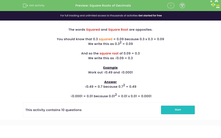# Find the Square Roots of Decimals

In this worksheet, students will state the square roots of decimal numbers.Key stage:  KS 3

Curriculum topic:   Number

Curriculum subtopic:   Understand Integer Powers/Real Roots

Popular topics:   Decimals worksheets

Difficulty level:#### Worksheet Overview

The words squared and square root are opposites.

We should know that 0.3 squared = 0.09 because 0.3 x 0.3 = 0.09

We write this as 0.32 = 0.09

And so the square root of 0.09 = 0.3

We write this as √0.09 = 0.3

Example

Work out √0.49 and √0.0001

√0.49 = 0.7 because 0.72 = 0.49

√0.0001 = 0.01 because 0.01= 0.01 x 0.01 = 0.0001

If you find some of these hard to do, remember to count the number of decimal places in the question and make sure that you have the same number of decimal places in the answer!√0.0001 has four decimal places, so we know that we must have four decimal places in the answer:

0.01 x 0.01 is four decimal places!

Time for some questions now.

### What is EdPlace?

We're your National Curriculum aligned online education content provider helping each child succeed in English, maths and science from year 1 to GCSE. With an EdPlace account you’ll be able to track and measure progress, helping each child achieve their best. We build confidence and attainment by personalising each child’s learning at a level that suits them.

Get started